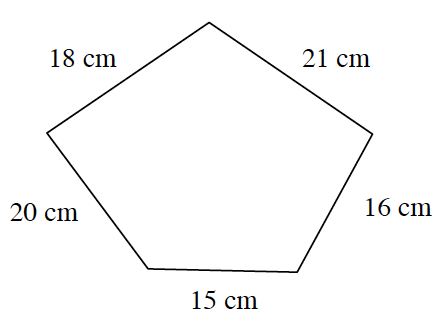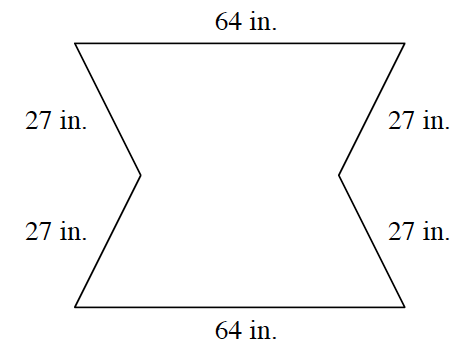### Home > MC1 > Chapter 2 > Lesson 2.1.4 > Problem2-47

2-47.

Find the perimeter (distance around) of each of the following figures.

1.The perimeter of a figure is equal to the sum of all the sides.

Add the lengths of all the sides together.

$18$ cm $+$ $20$ cm $+$ $15$ cm $+$ $16$ cm $+$ $21$ cm $=$ $90$ cm

The perimeter of this figure is $90$ cm.

1.This problem is done in the same way as part (a).